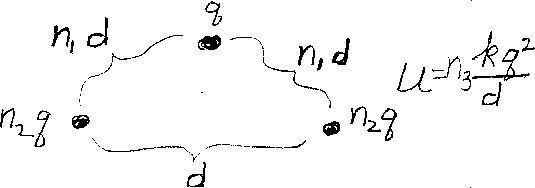Problem A13: What is the electrostatic energy of the three "point" objects shown in the figure above? Two objects have a charge of n2q and are separated by a distance of d. The third object has a charge of q and is separated by a distance of n1d from the other two. If the electrostatic energy is equal to U=n3 kq2/d, what is n3?n1 = n2 = Input n3:

If you are currently in my class, you can record your grade by entering your name and student ID number (without the leading zeros) below and clicking on "record grade".
 First Name = Last Name = ID = Problem: